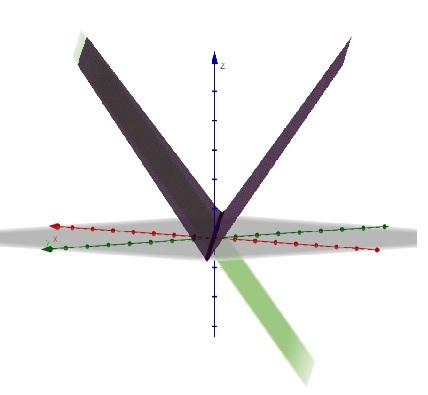# Find the sketch of z=f(x,y)= |x+y|.

## Question:

Find the sketch of {eq}z=f(x,y)= |x+y|{/eq}.

## 3D graph

We scetch the graph in 3D analogously as in 2D. Besides, we should be skilled in manipulating the equation of plane and its normal vector as also as the properties of an absolute value.

The picture is shown below. After that, there is step by step explanation.Firstly, we draw the graph of function

$$z=x+y$$

which is plane...

Become a Study.com member to unlock this answer! Create your account